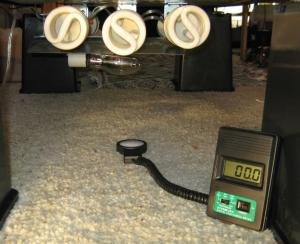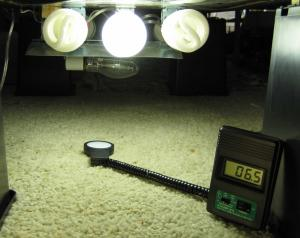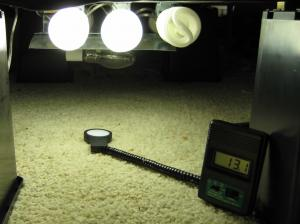GrowFAQ:

# What are lumens, lux, and all that?

 Added by: meatwad Rated by 0 users: 0.00/10 1. What is light? Light is electromagnetic radiation, and may be interpreted as either a wave or a particle: thus the phrase wave/particle duality. It exhibits phenomena that may be explained by its wavelike characteristics - interference, for example - while also demonstrating some that are best explained by its particle-like properties - such as the photoelectric effect. Treating light like a particle, a light source emits many light particles called photons. These are distinguishable by ONE unique property - their energy. When treating light like a wave, this is referred to as the wavelength, and is visible to us as a difference in color. The main point is this: photons of the same energy (wavelength, color) from different sources, for example MH, HPS, CFL, T5, etc. are THE SAME. 2. What is light intensity? Light intensity is simply the flux of photons per unit area per unit time. More photons means increased intensity. That's it. 3. What do lumens, lux, and foot-candles mean? *Lumen: This is the unit that defines "luminous flux", which is "radiant flux" (energy emission) adjusted for the sensitivity of the human eye. In the context of a light bulb, it is a measure of the total number of photons being emitted by the bulb (summed over all directions), adjusted for the sensitivity of the human eye to different photon energies. What this means is that different colors are weighted differently according to a "luminosity function" that describes the sensitivity of our eyes. You can see this function and a more detailed description here. *Lux, foot-candles: The lux is simply defined as the number of lumens per second incident upon one square meter. In other words, it is the number of photons striking a finite area per unit time, weighted by the luminosity function described above. The foot-candle is a derivative unit that is equal to 10.76 lux. It was defined based on the square foot, so the conversion is simply based on an area conversion. 4. What does this mean when using multiple light sources? So we've established that lux are the number of photons striking a unit area per unit time, weighted by a luminosity function. We've also established that photons from the same light source are indistinguishable, as long as they have the same energy/wavelength/color. What this means is that if you put two lights the same distance from a point, and each light provides N photons per unit area at the point, with two lights you will have 2N photons per unit area at the point. Because intensity is a measure of the number of photons per unit area, the light is twice as intense, whatever unit you choose to use. Twice the lumens, twice the lux, twice the footcandles. An obvious practical caveat to this point comes when using multiple low-intensity light sources. Notice how I stated that the lights were at the same distance? A practical problem with CFLs, for example, is that while you can get 27000 lumens from 10 x 42W CFLs, it's difficult to get them close enough to make them useful. If you have them in a line, for example, as I've shown below, each successive light is further from the meter, and the effective increase will be reduced. They still add, but according to the 1/d^2 rule, so having a bulb 2cm farther away will yield diminishing returns. On the other hand, this can be an effective way of distributing light, whereas with HID you need to distribute the plants around your single point light source. 5. Seriously? Prove it. Here is a simple experiment that demonstrates this point. Below are some 42W bulbs that are a part of a flowering cabinet. I have suspended it from some pots for the sake of this experiment. You can see there is also a 150W HPS in there; I'll do some HID vs. CFL comparisons at some point as well. The point is simple. Lights that are equidistant from a point contribute additively. Ambient light = 01 x 42W = 6500 ftc ~ 65000 lux2 x 42W = 13100 ftc ~ 131000 luxAny questions? More examples and experiments to come. Last modified: 06/2008
 Top of Page ©2009 - Cannabis Liberation Union (CLU)